Next: Moments of the Collision Up: Plasma Fluid Theory Previous: Introduction

# Moments of the Distribution Function

The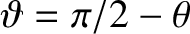th moment of the (ensemble averaged) distribution function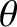is written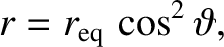(177)

withfactors of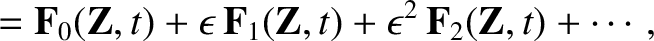. Clearly,is a tensor of rank.

The setcan be viewed as an alternative description of the distribution function, which, indeed, uniquely specifies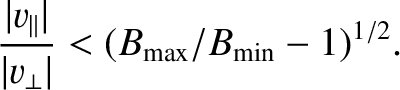when the latter is sufficiently smooth. For example, a (displaced) Gaussian distribution is uniquely specified by three moments: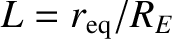, the vector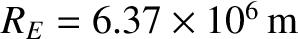, and the scalar formed by contracting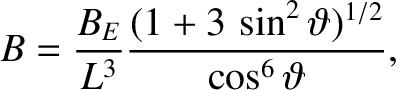.

The low-order moments all have names and simple physical interpretations. First, we have the (particle) density,(178)

and the particle flux density,(179)

The quantityis, of course, the flow velocity. Note that the electromagnetic sources, (166)-(167), are determined by these lowest moments: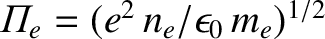(180)(181)

The second-order moment, describing the flow of momentum in the laboratory frame, is called the stress tensor, and denoted by(182)

Finally, there is an important third-order moment measuring the energy flux density,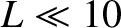(183)

It is often convenient to measure the second- and third-order moments in the rest-frame of the species under consideration. In this case, the moments assume different names: the stress tensor measured in the rest-frame is called the pressure tensor,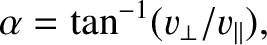, whereas the energy flux density becomes the heat flux density,. We introduce the relative velocity,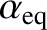(184)

in order to write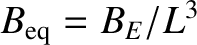(185)

and(186)

The trace of the pressure tensor measures the ordinary (or scalar'') pressure,(187)

Note thatis the kinetic energy density of species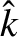: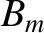(188)

In thermodynamic equilibrium, the distribution function becomes a Maxwellian characterized by some temperature, and Eq. (188) yields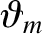. It is, therefore, natural to define the (kinetic) temperature as(189)

Of course, the moments measured in the two different frames are related. By direct substitution, it is easily verified that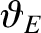(190)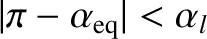(191)Next: Moments of the Collision Up: Plasma Fluid Theory Previous: Introduction
Richard Fitzpatrick 2011-03-31